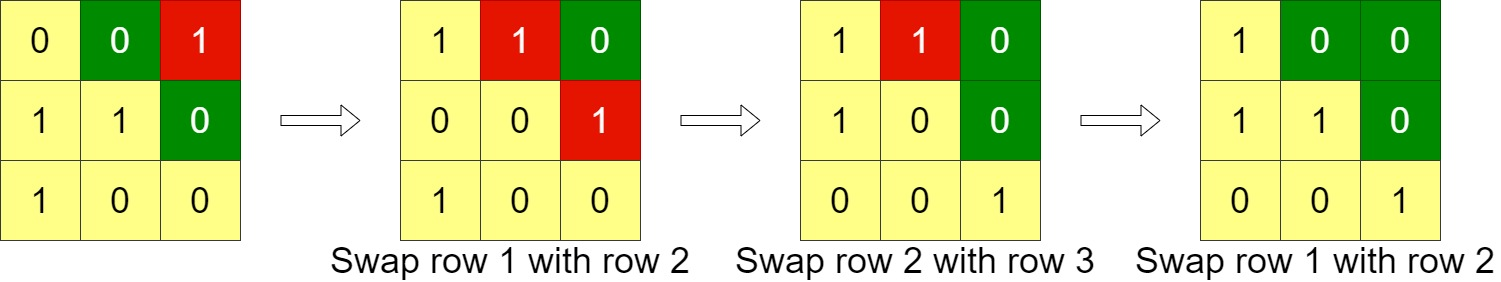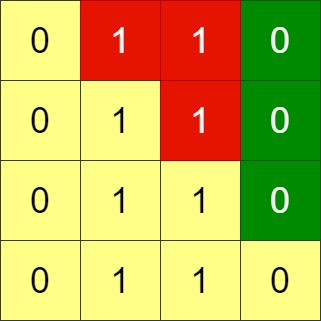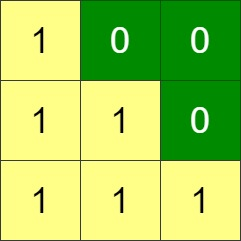# GeetCode Hub

Given an `n x n` binary `grid`, in one step you can choose two adjacent rows of the grid and swap them.

A grid is said to be valid if all the cells above the main diagonal are zeros.

Return the minimum number of steps needed to make the grid valid, or -1 if the grid cannot be valid.

The main diagonal of a grid is the diagonal that starts at cell `(1, 1)` and ends at cell `(n, n)`.

Example 1:```Input: grid = [[0,0,1],[1,1,0],[1,0,0]]
Output: 3
```

Example 2:```Input: grid = [[0,1,1,0],[0,1,1,0],[0,1,1,0],[0,1,1,0]]
Output: -1
Explanation: All rows are similar, swaps have no effect on the grid.
```

Example 3:```Input: grid = [[1,0,0],[1,1,0],[1,1,1]]
Output: 0
```

Constraints:

• `n == grid.length`
• `n == grid[i].length`
• `1 <= n <= 200`
• `grid[i][j]` is `0` or `1`

class Solution { public int minSwaps(int[][] grid) { } }